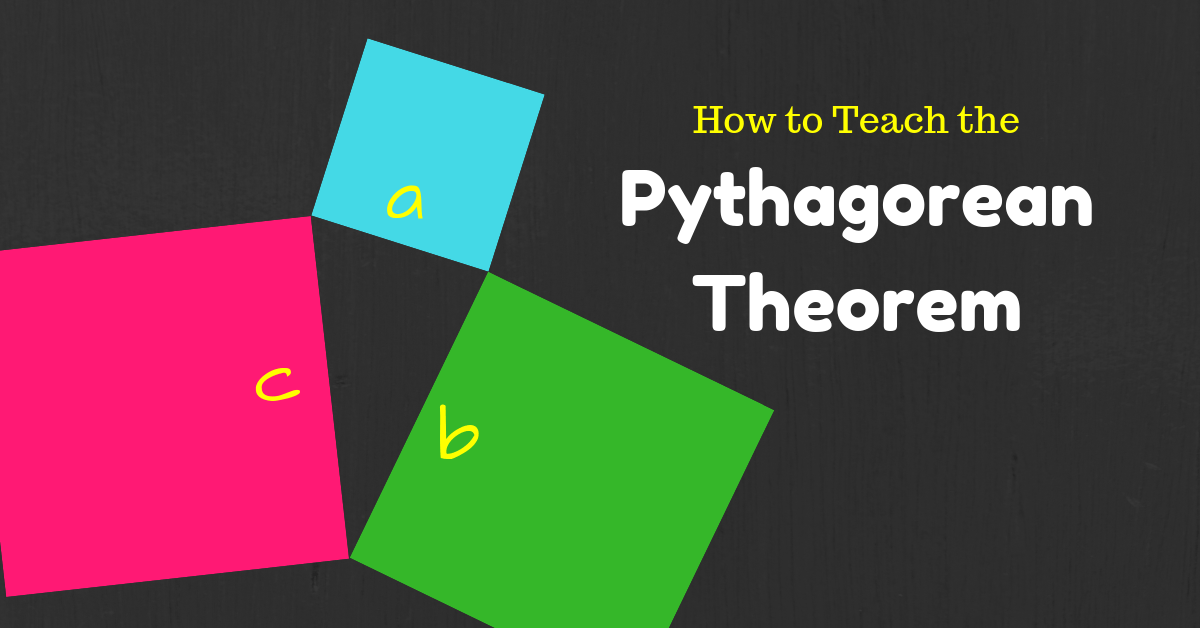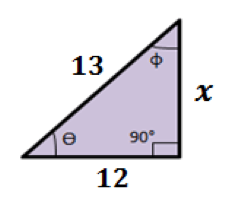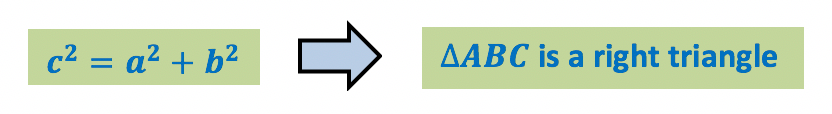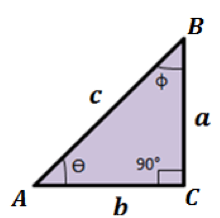# Pythagorean Theorem – NFL and Geometry

### “A Squared plus B Squared equals C Squared”

Over and over and over again. We pound it (The Pythagorean Theorem) into their young minds until it’s impossible that they forget it. There are two ways to teach this. It can be taught to the test and memorized with no recall as to when to use it only how to use it.The second option is to first let them discover WHEN to use the Pythagorean Theorem then teach them HOW to use. If you just draw them nice little pictures on the board to do these things you will lose them in 30 seconds. Start the lesson with a video like this one.

That will grab them and bring them into the discussion. Just think of the importance and impact of this lesson. If they do not clearly understand the properties of right triangles and the Pythagorean Theorem they can’t even begin to learn trigonometry. Do you have to show a football related video? No! There are plenty of videos on this topic that relate to all kinds of topics from designing a house to a dirt bike ramp.The bottom line is be creative don’t lose the class before you even start the lesson. This is one of the lessons in Geometry that has endless possibilities when it comes to creativity in the class room. Give them something memorable to recall so that when you teach them the Unit Circle they don’t have to memorize the whole thing because they lack these basic skills. For tips on teaching the Unit Circle: Click Here

## Pythagorean Theorem and its Converse Editable Materials

Lesson Plan

Bell Work Activity

Guided Notes

Vocabulary

Slide Show (PowerPoint Presentation)

Home Work Assignment

Online Activities

Video Lesson

Exit Quiz

## Here are your FREE Pythagorean Theorem Worksheet, Guided Notes, PowerPoint and More!

### PDFs

8-1 Assignment Teacher Edition – (Members Only)

8-1 Bell Work Teacher Edition – (Members Only)

8-1 Exit Quiz Teacher Edition – (Members Only)

8-1 Guided Notes Teacher Edition – (Members Only)

8-1 Lesson Plan (Members Only)

8-1 Video Lesson (Members Only)

8-1 Online Activities (Members Only)

### Word Docs & PowerPoints

To gain access to our editable content for Parallel Lines and Transversals Join the Geometry Teacher Community!
Here you will find hundreds of lessons, a community of teachers for support, and materials that are always up to date with the latest standards.

Instead of using every free moment you get to research Geometry Lesson Plans, creating material for your classroom, and stressing every night over getting your lesson done let us do all the work for you! Get your life back, spend time with your family, NEVER CREATE A LESSON PLAN AGAIN! Join our Geometry Teacher Community Now!! Click the image below to get access to the rest of our materials on Parallel Lines and Transversals.## Pythagorean Theorem and its Converse Guided Notes

A Right-Angled Triangle (named as right triangle) is a triangle which has one of its angles equal to 90 degrees.### There are properties associated with a right triangle.• A Hypotenuse is the line segment opposite to the right-angle. The Hypotenuse is also the longest side of a Right Triangle.
• An Opposite is the line segment opposite to the angle ϴ.
• An Adjacent is the line segment next to the angle ϴ.
• The sum of three angles is 180°
e. ϴ + φ + 90° = 180°

### Pythagorean Theorem

In a Right Triangle, the sum of the squares of the lengths of adjacent and opposite side is equal to the square of the length of hypotenuse.

a2+b2=c2

Where,c= Hypotenuse

a= Opposite

### Problem 1: Find the unknown length in the right triangle shown.

By Pythagorean theorem,

c2=a2+b2This means that,

132=x2+122

x2=169-144

x2=25

x=5

### Converse of Pythagorean Theorem

If the sum of the squares of the lengths of adjacent and opposite sides is equal to the square of the length of hypotenuse, then the triangle is a right triangle.Where,c= Hypotenuse

a= Opposite

### Problem 2: Identify if the triangle shown is a right triangle or not.

To show if the triangle is a right triangle, we need to check whether its lengths satisfy the Pythagorean theorem:

c2=a2+b2If this triangle is right then this also must be true,

102=82+62

100=64+36

100=100

So, the triangle is a right triangle.

## Another Super Engaging Pythagorean Theorem Lesson:

If you are still struggling to get your students engaged with your Pythagorean Theorem Lesson we have another lesson available on PreAlgebraCoach.com. The lesson teaches the students to derive the Pythagorean Theorem on their own rather than just giving it to them and then some sample problems. The lesson below is an extremely engaging way to teach the Pythagorean Theorem and its Converse. I would recommend teaching it this way then go through the above material.

Here is the Link to Access the Lesson:

https://prealgebracoach.com/the-pythagorean-theorem/

This lesson is based around creating (Deriving) the Pythagorean Theorem together in class using GOLF!